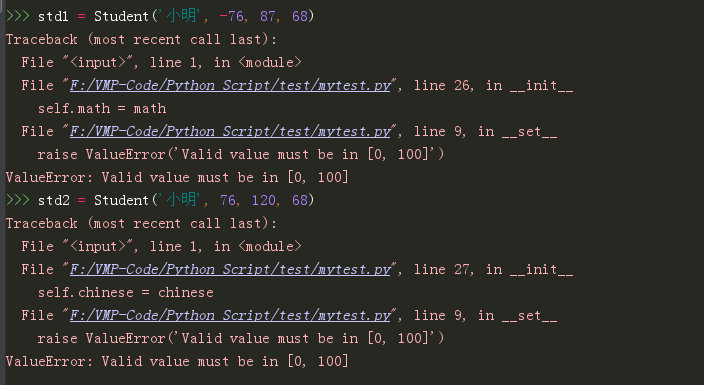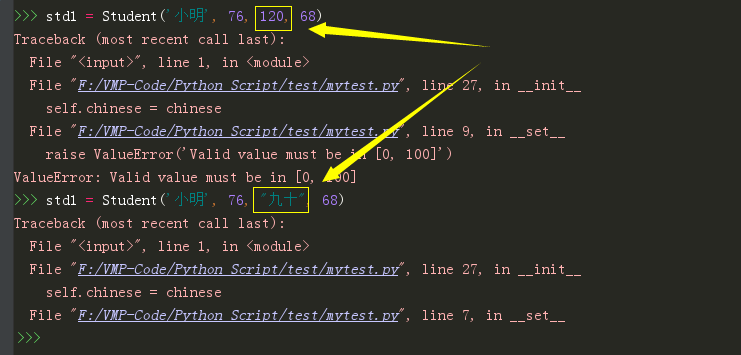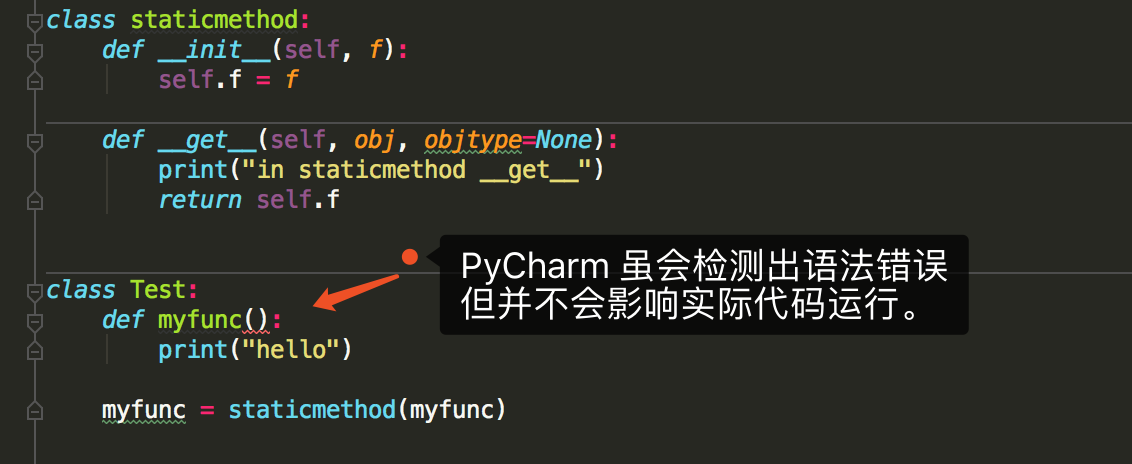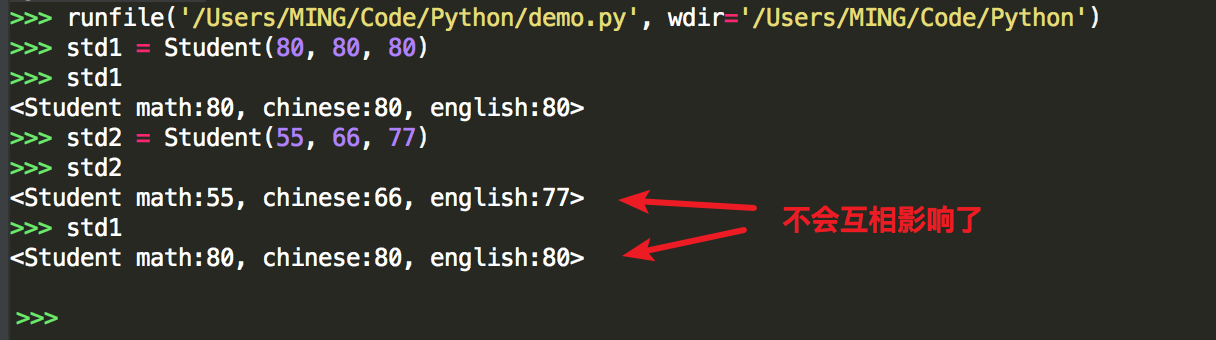# 7.11 【进阶】深藏不露的描述符（Descriptor）¶

## 1. 为什么要使用描述符？¶

```class Student:
def __init__(self, name, math, chinese, english):
self.name = name
self.math = math
self.chinese = chinese
self.english = english

def __repr__(self):
return "<Student: {}, math:{}, chinese: {}, english:{}>".format(
self.name, self.math, self.chinese, self.english
)
```

```>>> std1 = Student('小明', 76, 87, 68)
>>> std1
<Student: 小明, math:76, chinese: 87, english:68>
```

```class Student:
def __init__(self, name, math, chinese, english):
self.name = name
if 0 <= math <= 100:
self.math = math
else:
raise ValueError("Valid value must be in [0, 100]")

if 0 <= chinese <= 100:
self.chinese = chinese
else:
raise ValueError("Valid value must be in [0, 100]")

if 0 <= chinese <= 100:
self.english = english
else:
raise ValueError("Valid value must be in [0, 100]")

def __repr__(self):
return "<Student: {}, math:{}, chinese: {}, english:{}>".format(
self.name, self.math, self.chinese, self.english
)
``````class Student:
def __init__(self, name, math, chinese, english):
self.name = name
self.math = math
self.chinese = chinese
self.english = english

@property
def math(self):
return self._math

@math.setter
def math(self, value):
if 0 <= value <= 100:
self._math = value
else:
raise ValueError("Valid value must be in [0, 100]")

@property
def chinese(self):
return self._chinese

@chinese.setter
def chinese(self, value):
if 0 <= value <= 100:
self._chinese = value
else:
raise ValueError("Valid value must be in [0, 100]")

@property
def english(self):
return self._english

@english.setter
def english(self, value):
if 0 <= value <= 100:
self._english = value
else:
raise ValueError("Valid value must be in [0, 100]")

def __repr__(self):
return "<Student: {}, math:{}, chinese: {}, english:{}>".format(
self.name, self.math, self.chinese, self.english
)
```• `__get__`： 用于访问属性。它返回属性的值，若属性不存在、不合法等都可以抛出对应的异常。

• `__set__`：将在属性分配操作中调用。不会返回任何内容。

• `__delete__`：控制删除操作。不会返回内容。

```class Score:
def __init__(self, default=0):
self._score = default

def __set__(self, instance, value):
if not isinstance(value, int):
raise TypeError('Score must be integer')
if not 0 <= value <= 100:
raise ValueError('Valid value must be in [0, 100]')

self._score = value

def __get__(self, instance, owner):
return self._score

def __delete__(self):
del self._score

class Student:
math = Score(0)
chinese = Score(0)
english = Score(0)

def __init__(self, name, math, chinese, english):
self.name = name
self.math = math
self.chinese = chinese
self.english = english

def __repr__(self):
return "<Student: {}, math:{}, chinese: {}, english:{}>".format(
self.name, self.math, self.chinese, self.english
)
```## 2. 描述符的访问规则¶

• 数据描述符：实现了`__get__``__set__` 两种方法的描述符

• 非数据描述符：只实现了`__get__` 一种方法的描述符

```# 数据描述符
def __init__(self, default=0):
self._score = default

def __set__(self, instance, value):
self._score = value

def __get__(self, instance, owner):
print("访问数据描述符里的 __get__")
return self._score

# 非数据描述符
def __init__(self, default=0):
self._score = default

def __get__(self, instance, owner):
print("访问非数据描述符里的 __get__")
return self._score

class Student:

def __init__(self, name, math, chinese):
self.name = name
self.math = math
self.chinese = chinese

def __getattribute__(self, item):
print("调用 __getattribute__")
return super(Student, self).__getattribute__(item)

def __repr__(self):
return "<Student: {}, math:{}, chinese: {},>".format(
self.name, self.math, self.chinese)
```

```>>> std = Student('xm', 88, 99)
>>>
>>> std.math

88
>>> std.chinese

99
```

## 3. 基于描述符如何实现property¶

```class Student:
def __init__(self, name):
self.name = name

@property
def math(self):
return self._math

@math.setter
def math(self, value):
if 0 <= value <= 100:
self._math = value
else:
raise ValueError("Valid value must be in [0, 100]")
```

```class TestProperty(object):

def __init__(self, fget=None, fset=None, fdel=None, doc=None):
self.fget = fget
self.fset = fset
self.fdel = fdel
self.__doc__ = doc

def __get__(self, obj, objtype=None):
print("in __get__")
if obj is None:
return self
if self.fget is None:
raise AttributeError
return self.fget(obj)

def __set__(self, obj, value):
print("in __set__")
if self.fset is None:
raise AttributeError
self.fset(obj, value)

def __delete__(self, obj):
print("in __delete__")
if self.fdel is None:
raise AttributeError
self.fdel(obj)

def getter(self, fget):
print("in getter")
return type(self)(fget, self.fset, self.fdel, self.__doc__)

def setter(self, fset):
print("in setter")
return type(self)(self.fget, fset, self.fdel, self.__doc__)

def deleter(self, fdel):
print("in deleter")
return type(self)(self.fget, self.fset, fdel, self.__doc__)
```

```class Student:
def __init__(self, name):
self.name = name

# 其实只有这里改变
@TestProperty
def math(self):
return self._math

@math.setter
def math(self, value):
if 0 <= value <= 100:
self._math = value
else:
raise ValueError("Valid value must be in [0, 100]")
```

1. 使用`TestProperty`装饰后，`math` 不再是一个函数，而是`TestProperty` 类的一个实例。所以第二个math函数可以使用 `math.setter` 来装饰，本质是调用`TestProperty.setter` 来产生一个新的 `TestProperty` 实例赋值给第二个`math`

2. 第一个 `math` 和第二个 `math` 是两个不同 `TestProperty` 实例。但他们都属于同一个描述符类（TestProperty），当对 math 对于赋值时，就会进入 `TestProperty.__set__`，当对math 进行取值里，就会进入 `TestProperty.__get__`。仔细一看，其实最终访问的还是Student实例的 `_math` 属性。

```# 运行后，会直接打印这一行，这是在实例化 TestProperty 并赋值给第二个math
in setter
>>>
>>> s1.math = 90
in __set__
>>> s1.math
in __get__
90
```

## 4. 基于描述符如何实现staticmethod¶

```class Test:
@staticmethod
def myfunc():
print("hello")

# 上下两种写法等价

class Test:
def myfunc():
print("hello")
# 重点：这就是描述符的体现
myfunc = staticmethod(myfunc)
```

```@TestProperty
def math(self):
return self._math

math = TestProperty(fget=math)
``````>>> Test.myfunc()
in staticmethod __get__
hello
>>> Test().myfunc()
in staticmethod __get__
hello
```

## 5. 基于描述符如何实现classmethod¶

```class classmethod(object):
def __init__(self, f):
self.f = f

def __get__(self, instance, owner=None):
print("in classmethod __get__")

def newfunc(*args):
return self.f(owner, *args)
return newfunc

class Test:
def myfunc(cls):
print("hello")

# 重点：这就是描述符的体现
myfunc = classmethod(myfunc)
```

```>>> Test.myfunc()
in classmethod __get__
hello
>>> Test().myfunc()
in classmethod __get__
hello
```

## 6. 所有实例共享描述符¶

```class Score:
def __init__(self, default=0):
self._value = default

def __get__(self, instance, owner):
return self._value

def __set__(self, instance, value):
if 0 <= value <= 100:
self._value = value
else:
raise ValueError

class Student:
math = Score(0)
chinese = Score(0)
english = Score(0)

def __repr__(self):
return "<Student math:{}, chinese:{}, english:{}>".format(self.math, self.chinese, self.english)
```

Student 里没有像前面那样写了构造函数，但是关键不在这儿，没写只是因为没必要写。

```>>> std1 = Student()
>>> std1
<Student math:0, chinese:0, english:0>
>>> std1.math = 85
>>> std1
<Student math:85, chinese:0, english:0>
>>> std2 = Student()
>>> std2 # std2 居然共享了std1 的属性值
<Student math:85, chinese:0, english:0>
>>> std2.math = 100
>>> std1 # std2 也会改变std1 的属性值
<Student math:100, chinese:0, english:0>
```

```class Score:
def __init__(self, subject):
self.name = subject

def __get__(self, instance, owner):
return instance.__dict__[self.name]

def __set__(self, instance, value):
if 0 <= value <= 100:
instance.__dict__[self.name] = value
else:
raise ValueError

class Student:
math = Score("math")
chinese = Score("chinese")
english = Score("english")

def __init__(self, math, chinese, english):
self.math = math
self.chinese = chinese
self.english = english

def __repr__(self):
return "<Student math:{}, chinese:{}, english:{}>".format(self.math, self.chinese, self.english)
```• 之前的错误代码，更像是把描述符当做了存储节点。

• 之后的正确代码，则是把描述符直接当做代理，本身不存储值。